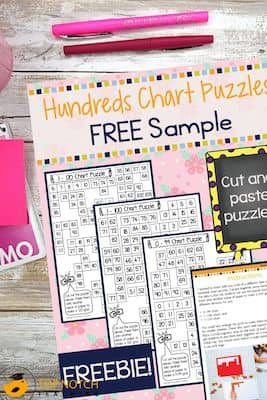# 6 Great Ideas For Teaching Place Value

Teaching place value is important for students. Place value helps us to read whole numbers into the millions and beyond. Try these place value activities.Getting clear on place value is important for students. Place value helps us to read whole numbers into the thousands, millions, billions, and beyond. Do your students need more practice with this pattern we use to put numbers together? Try these place value activities.

## Activities for teaching place value

1. Use manipulatives for teaching place value. Give students a number. Have them create the number using Unifix cubes, number blocks, or other objects. For example, if you said 528, students would select 5 hundreds blocks, 2 tens blocks, and 8 ones blocks. Have students put the blocks in the right order (hundreds, tens, ones).

Alternately, you can create a number using number blocks. Have students say and write the number it represents.2. Use place value charts when teaching place value. Place value charts are an obvious tool for teaching place value, but what do you do with them? Try:

—Reading a number aloud and having students record it in the chart.
—Writing a number in digits and having students record it in the chart.
—Give students a number in words and have them record the correct digits in the chart.
—Give students two numbers and have them record the larger (or smaller number) in the chart.
—Give students two numbers and have them record the number with the larger number in the tens place (or hundreds or thousands) in the chart.

These activities help students first identify which number goes in each place. Then they have students compare numbers using place value as well.3. Use number lines in place value activities. Give students a number line with some numbers filled in. Give students several numbers to add in the correct place to the number line. Students will need to pay attention to place value to determine where each number belongs.

You can also give each student a number (base the numbers on the highest place value you are working with. For example, if you are working on thousands, use numbers like 1346, 1463, and 1634). Have the students create a large number line by putting themselves in order smallest to largest.

4. Use hundreds charts for place value activities. Hundreds charts that go beyond 100 are a great tool to use for understanding place value. Ask students to look for patterns in columns (ones place will be the same) or in the rows (tens place will be the same).Number charts that go beyond 100 are a great tool to use helping students overcome skip counting barriers when they reach a place value barrier. To help them get past that place value and recognize a new place value, have students start by counting out 10 on their hundreds chart. Let them count out ten a few times. Then ask them if they see a pattern. They may notice that all the answers end in 0 or that they are all in the same column. Then ask them to predict the next few numbers by looking at the chart without counting it out. Having the visual on the chart helps them see what’s next. Try this with other numbers.

Give students additional practice with place values and hundreds charts with these puzzles. Students put the puzzles together and then can use the hundreds charts for these activities and others.

### FREE Hundreds Chart Puzzles5. Use place value mats for teaching place value. When you laminate your place value mats, you can use them in a number of ways. You can use it with whiteboard markers as a reusable place value chart. You can use it as a play dough mat and have students form numbers out of dough to fill in the chart. You can have students use blocks or other items to fill in the different place values in the mat.

6. Use number expanders for place value activities. Number expanders are a fun way for students to show numbers. First talk with students about different ways to expand numbers. For example, 268 could be:

—268 ones
—26 tens and 8 ones
—2 hundreds, 6 tens, and 8 ones

Then have students make a number expander tool You’ll need long strips of paper. You can make them for as many place values as you like. Each expander needs space for a number followed by the place value label, another number and the next place value label, all the way down to one. Students write in the correct numbers and fold to show the number given.

Need more place value activities? You’ll love these place value task cards for building number sense: https://topnotchteaching.com/downloads/place-value-cards/### FREE Hundreds Chart Puzzles## 1 Comment

1.Thank you for the wonderful teaching place value ideas! I’ll definitely try the hundreds charts.